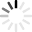# Polygon Quizzes & Trivia

What do you get when you take a word meaning "many" and another word meaning "side"? You get a two or three dimensional object that can be used to build anything from the answer in an exam, to a house! It's a polygon! What exactly is a polygon? Do you know, or is the answer "gon" from your mind?

With hexagons and pentagons, cubes and the theorectical monogon, your inner-geometricist will have a cube (as a ball isn't a polygon, you can't have one of those) with this quiz! Can you answer why a monogon is impossible? Why the triangle is the favorite shape to be used by architects? or what a nine-sided polygon is called? Yes? Then why are you standing on the side(s)? Prepare to leave your current dimension and join the revolution!
Top Trending

Questions: 10  |  Attempts: 11903   |  Last updated: Oct 11, 2017
• Sample Question
How many sides does a pentagon have?Questions: 20  |  Attempts: 673   |  Last updated: Dec 21, 2020
• Sample Question
Squares are always rectangles.When finding the angles of a polygon, we usually divide the polygon into triangles. There is a basic formula for getting the angles that was covered in class today. Take up the quiz below to help you practice on getting the...

Questions: 10  |  Attempts: 803   |  Last updated: Nov 18, 2020
• Sample Question
What is the interior angle sum of a decagon?Polygons are shapes with two or more sides. They are made from lines that are joined together at certain angles. For a regular polygon, there are at least two sides that fuse to form a vertex. Some polygons have equal, interior...

Questions: 10  |  Attempts: 459   |  Last updated: Apr 8, 2019
• Sample Question
What is a polygon with five sides called?A polygon is a geometrical plane figure bounded by a chain of straight line segments which close in a loop to form a two-dimensional shape. Examples include squares, triangles and rectangles. What do you know about them?

Questions: 6  |  Attempts: 323   |  Last updated: Apr 27, 2021TopicsRelated